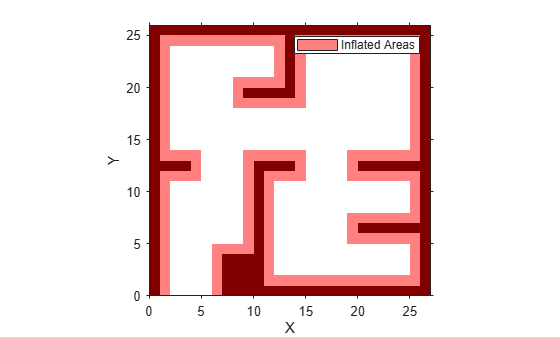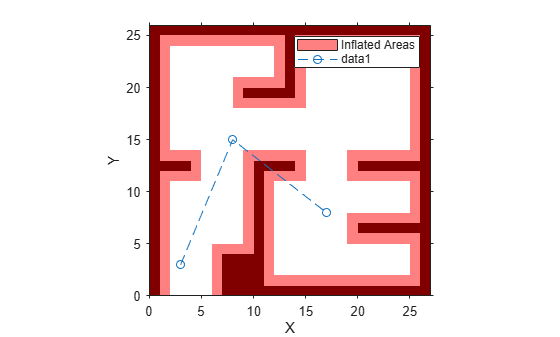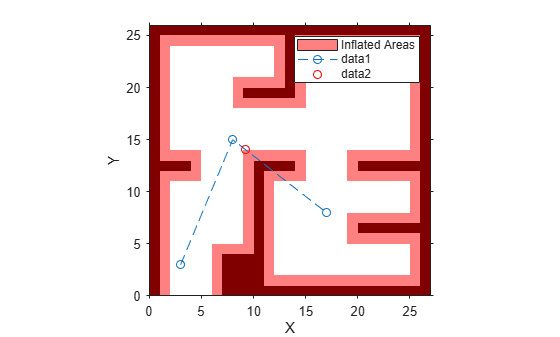# validatorVehicleCostmap

State validator based on 2-D costmap

## Description

The `validatorOccupancyMap` object validates states and discretized motions based on the value in a 2-D costmap. An occupied map location is interpreted as an invalid state.

## Creation

### Description

````validator = validatorVehicleCostmap` creates a vehicle cost map validator associated with an SE2 state space with default settings.```

example

````validator = validatorVehicleCostmap(stateSpace)` creates a validator in the given state space definition derived from `nav.StateSpace`.```
````validator = validatorVehicleCostmap(stateSpace,xyIndices)`sets the `XYIndices` property to specify which variables in the state vector define the xy-coordinates.```
````validator = validatorVehicleCostmap(stateSpace,Name,Value)` specifies the `Map` or `XYIndices` properties using `Name,Value` pair arguments.```

## Properties

expand all

State space for validating states, specified as a subclass of `nav.StateSpace`. Provided state space objects include:

Map used for validating states, specified as a `vehicleCostmap` (Automated Driving Toolbox) object.

Interval for sampling between states and checking state validity, specified as a positive numeric scalar.

State variable mapping for xy-coordinates in state vector, specified as a two-element vector, `[xIdx yIdx]`. For example, if a state vector is given as `[r p y x y z]`, the xy-coordinates are `[4 5]`.

State variable mapping for theta coordinate in state vector, specified as a positive integer. For example, if a state vector is given as ```[x y theta]```, the theta coordinate is `3`.

## Object Functions

 `copy` Create deep copy of state validator object `isStateValid` Check if state is valid `isMotionValid` Check if path between states is valid

## Examples

collapse all

This example shows how to validate paths through an evironment.

Load example maps. Use the simple map to create a vehicle cost map. Specify an inflation raidus of 1 meter.

```load exampleMaps.mat map = vehicleCostmap(double(simpleMap)); map.CollisionChecker = inflationCollisionChecker("InflationRadius",1); plot(map)```Specify a coarse path through the map.

```path = [3 3 pi/2; 8 15 0; 17 8 -pi/2]; hold on plot(path(:,1),path(:,2),"--o")```Create a state validator using the `stateSpaceSE2` definition. Specify the map and the distance for interpolating and validating path segments.

```validator = validatorVehicleCostmap(stateSpaceSE2); validator.Map = map; validator.ValidationDistance = 0.1;```

Check the points of the path are valid states. All three points are in free space, so are considered valid.

`isValid = isStateValid(validator,path)`
```isValid = 3x1 logical array 1 1 1 ```

Check the motion between each sequential path states. The `isMotionValid` function interpolates along the path between states. If a path segment is invalid, plot the last valid point along the path.

```startStates = [path(1,:);path(2,:)]; endStates = [path(2,:);path(3,:)]; for i = 1:2 [isPathValid, lastValid] = isMotionValid(validator,startStates(i,:),endStates(i,:)); if ~isPathValid plot(lastValid(1),lastValid(2),'or') end end hold off```## Version History

Introduced in R2019b